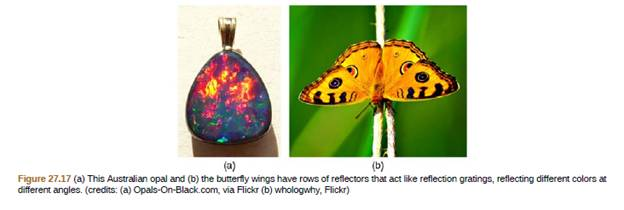Chapter 27, Problem 32PE

Chapter
Section
Textbook Problem

An opal such as that shown in Figure 27.17 acts like a reflection grating with rows separated by about 8 μ m. If the opal is illuminated normally, (a) at what angle will red light be seen and (b) at what angle will blue light be seen?To determine

(a)

At what angle will red light be seen?

Explanation

Given info:

Figure 27.17 acts like reflecting grating with rows separated by about 8μm . The opal is illuminated normally.

Formula used:

Constructive interference of light from rows in crystal is

dsinθ=mλ

Here, d is the distance between slits, λ is the wavelength of light, m is the order of the maximum and θ is the angle from the original direction of the beam.

Calculation:

We have, d=8×106m, λ=700 nm, m=1.

Substituting these values in above, relation we get

(8× 10 6m)sinθ=1×7

To determine

(b)

At what angle will blue light be seen?

Still sussing out bartleby?

Check out a sample textbook solution.

See a sample solution

The Solution to Your Study Problems

Bartleby provides explanations to thousands of textbook problems written by our experts, many with advanced degrees!

Get Started

Find more solutions based on key concepts